# Banked Curve-Determine smallest static coefficient of friction

## Homework Statement

13.49 The flatbed railway car travels at the constant speed of 80 km/h around a curve
of radius 55m and the bank angle 15deg. Determine the smallest static coefficient of friction between the crate and the car that would prevent the crate of mass M from sliding.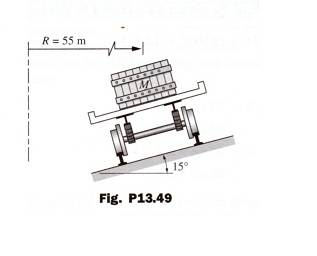## The Attempt at a Solution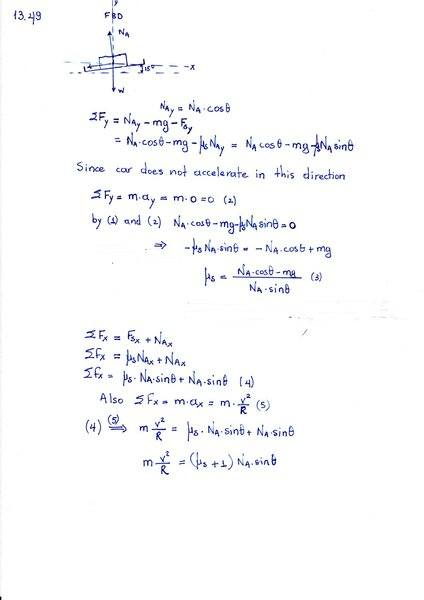[/B]
Am I at the right track? If yes how do I proceed?

kuruman
Homework Helper
Gold Member
It looks like you got confused with the algebra. You cannot have μs and NA together in your final expression. It might be cleaner to use a coordinate system where the x-axis is aligned with the incline. Then the normal force and the force of static friction are in separate equations. Find an expression for NA from the y-equation and put it in the y-equation in μsNA. Don't forget that the acceleration has both x and y components in this system of axes.

•TSny
TSny
Homework Helper
Gold Member
kuruman's suggestion is good. If you decide to stay with your orientation of axes, you will need to correct the following: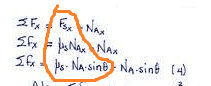The horizontal component of friction does not equal μs times the horizontal component of the normal force.

kuruman
Homework Helper
Gold Member
One more thing you need to consider is the direction of the force of friction. For a given coefficient of friction, radius of curvature and banking angle there is a range of speeds that will keep the car on track. How do you know that it is towards the center of the circle? If the car goes "too fast", yes the force of friction is towards the center, but if the car goes "too slow", friction needs to point away from the center. In short, how do you determine whether the speed is too fast or too slow?

kuruman's suggestion is good. If you decide to stay with your orientation of axes, you will need to correct the following:
View attachment 211960

The horizontal component of friction does not equal μs times the horizontal component of the normal force.

Ah, yes I got it wrong! It should be ##Fs_x = F_s cosθ = μ_s N_A cosθ##

Also in response to kuruman: I don't know how to determine whether the speed is too fast or too slow

My thought is if the railway car travels at the constant speed of 80Km/h around a the curve then the crate will have the tendency to move away from the center of the circle causing a friction force pointing to the center of the circle.

Last edited:
kuruman
Homework Helper
Gold Member
My thought is if the railway car travels at the constant speed of 80Km/h around a the curve then the crate will have the tendency to move away from the center of the circle causing a friction force pointing to the center of the circle.
Suppose the angle of the incline were 90o and the car has enough speed as it goes around to stay at fixed height (daredevils on motorcycles do this all the time.) Then surely fs must point up the incline to cancel the weight. Assuming no sliding at any time, as you decrease the angle keeping the speed constant, fs will become smaller, go through zero and then change direction. The critical angle is matched to the particular speed. Now suppose you fix the angle and change the speed. There is a critical speed at that angle at which the friction is zero. Can you find the critical speed for this problem? That's what determines whether the given speed is too fast or too slow and hence the direction of friction.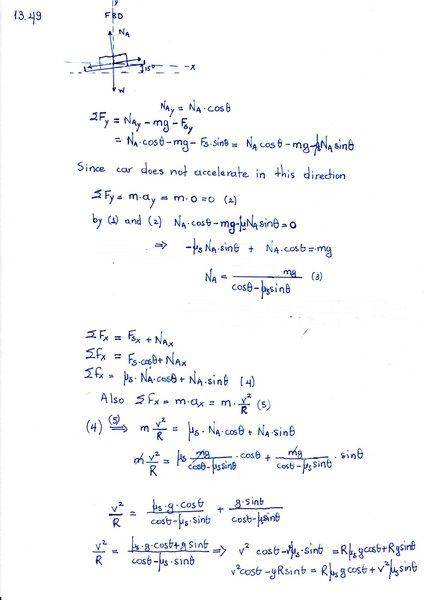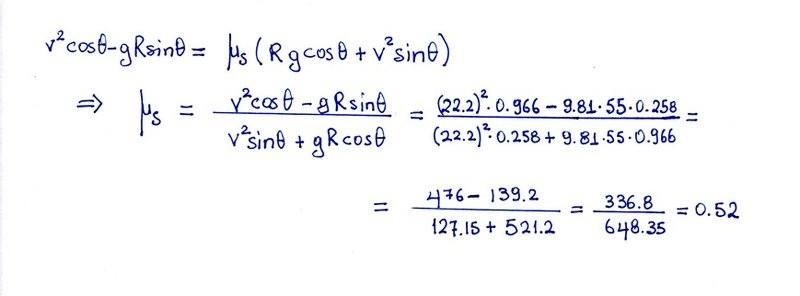Suppose the angle of the incline were 90o and the car has enough speed as it goes around to stay at fixed height (daredevils on motorcycles do this all the time.) Then surely fs must point up the incline to cancel the weight. Assuming no sliding at any time, as you decrease the angle keeping the speed constant, fs will become smaller, go through zero and then change direction. The critical angle is matched to the particular speed. Now suppose you fix the angle and change the speed. There is a critical speed at that angle at which the friction is zero. Can you find the critical speed for this problem? That's what determines whether the given speed is too fast or too slow and hence the direction of friction.

Doing some research on the web regarding the problem in question I found two good sources of info:
1)https://physics.stackexchange.com/q...-find-direction-of-friction-on-a-banked-curve

According to the above the critical speed can be found when the frictional force equals zero so that the component of
W parallel to the slope is equalthe centripetal force.
so ## Wsinθ=ma_c## => ## mgsinθ=m\frac {v^2} {r}## => ##v = \sqrt {gsinθr} = 11.82 m/s ##

Since the speed of the car is bigger than the critical speed the friction force should be parallel to the slope
to the left (towards the center of curve).

kuruman
Homework Helper
Gold Member
Your expression for μs is correct. Your expression for the critical speed is not. If μs = 0 (frictionless case), then we v = vcrit.. Your expression then gives ##v^2_{crit.}\cos \theta-g R \sin \theta=0##. If you solve for ##v_{crit.}##, what do you get?

Your expression for μs is correct. Your expression for the critical speed is not. If μs = 0 (frictionless case), then we v = vcrit.. Your expression then gives ##v^2_{crit.}\cos \theta-g R \sin \theta=0##. If you solve for ##v_{crit.}##, what do you get?
Ah, yes.
It should be ##v^2_{crit} cosθ - gRsinθ = 0## => ##v^2_{crit}=\frac {gRsinθ} {cosθ}## => ##v_{crit} = \sqrt {gRtanθ}## => ##v_{crit} = 12.02 \frac {m} {s}##

So my result 0.52 for ##μ_s## is correct?

haruspex